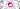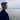# 用 RxJS 连接世界

November 06, 2016## 准备工作

1. 按回车或点击 add button 后发送一个请求，在请求返回结果后再清空输入框，并将返回的结果变成一个 todo item。如果在请求返回结果前又一次按下回车或 add 按钮，对比此时输入框的值和上次发送的值是否相同，如果相同则不进行任何操作，如果不同则取消掉上次的请求并发送新的请求。
2. 在点击一个 todo item 的时候发送请求，间隔 300 毫秒内的点击，只会发出一次请求。
3. 在输入框中每次输入字符，都会在 200 毫秒后发送一个请求，搜索是否匹配到已存在的 todo item，如果已存在则高亮这个 todo item。如果在一次搜索的结果返回前输入了新的字符，则取消掉前一个请求，再发一个搜索请求。

## 使用 switchMap 切换 Observable

``````export const mockHttpPost = (value: string): Observable<HttpResponse> => {
return Observable.create((observer: Observer<HttpResponse>) => {
let status = 'pending'
const timmer = setTimeout(() => {
const result = {
_id: ++dbIndex,
value,
isDone: false,
}
searchStorage.set(result._id, result)
status = 'done'
observer.next(result)
observer.complete()
}, random(10, 1000))
return () => {
clearTimeout(timmer)
if (status === 'pending') {
console.warn('post canceled')
}
}
})
}``````

``````Observable.create((observer) => {
request(
xxxx,
(response) => {
// success callback
observer.next(parse(response))
observer.complete()
},
(err) => {
// error callback
observer.error(err)
},
)
// teardown logic
return () => request.abort()
})``````

app.ts 中引入 `mockHttpPost`:

``````...
import {
createTodoItem,
mockHttpPost
} from './lib'

...

const item\$ = input\$
.map(() => \$input.value)
.filter(r => r !== '')
.switchMap(mockHttpPost)
.map(data => createTodoItem(data.value))
.do((ele: HTMLLIElement) => {
\$list.appendChild(ele)
\$input.value = ''
})
.publishReplay(1)
.refCount()``````

``````// lib.ts
export const createTodoItem = (data: HttpResponse) => {
const result = <HTMLLIElement>document.createElement('LI')
result.classList.add('list-group-item', `todo-item-\${data._id}`)
result.setAttribute('data-id', `\${data._id}`)
const innerHTML = `
\${data.value}
<button type="button" class="btn btn-default button-remove pull-right" aria-label="right Align">
<span class="glyphicon glyphicon-remove" aria-hidden="true"></span>
</button>
`
result.innerHTML = innerHTML
return result
}``````

``````const item\$ = input\$
.map(() => \$input.value)
.filter((r) => r !== '')
.switchMap(mockHttpPost)
.map(createTodoItem)
.do((ele: HTMLLIElement) => {
\$list.appendChild(ele)
\$input.value = ''
})
.publishReplay(1)
.refCount()``````

1. 直接输入值并回车，todo item 像以前一样被创建
2. 输入值，并在 todo item 生成前多次回车，可以看到请求被 cancel 了多次:

``````const item\$ = input\$
.map(() => \$input.value)
.filter(r => r !== '')
.map(mockHttpPost)
.switch()
.map(createTodoItem)
...``````

``````// 你可以在项目目录下执行: npm i -g ts-node && ts-node example/switchMap.ts 观察运行结果
import { Observable, Observer } from 'rxjs'

const stream = Observable.create((observer: Observer<number>) => {
let i = 0
const intervalId = setInterval(() => {
observer.next(++i)
}, 1000)
return () => clearInterval(intervalId)
})

function createIntervalObservable(base: number) {
let i = 0
return Observable.create((observer: Observer<string>) => {
const intervalId = setInterval(() => {
observer.next(`base: \${base}, value: \${++i}`)
}, 200)
return () => {
clearInterval(intervalId)
console.log(`unsubscribe base: \${base}`)
}
})
}

stream
.switchMap(createIntervalObservable)
.subscribe((result) => console.log(result))``````

## 使用 distinct* 操作符过滤数据

``````const item\$ = input\$
.map(() => \$input.value)
.filter((r) => r !== '')
.distinct()
.switchMap(mockHttpPost)
.map(createTodoItem)
.do((ele: HTMLLIElement) => {
\$list.appendChild(ele)
\$input.value = ''
})
.publishReplay(1)
.refCount()``````

## 使用 Subject 推送数据

``````import { Observable, Subject } from 'rxjs'
...

const clearInputSubject\$ = new Subject<void>()

const item\$ = input\$
.map(() => \$input.value)
.filter(r => r !== '')
.distinct(null, clearInputSubject\$)
.switchMap(mockHttpPost)
.map(createTodoItem)
.do((ele: HTMLLIElement) => {
\$list.appendChild(ele)
\$input.value = ''
clearInputSubject\$.next()
})
.publishReplay(1)
.refCount()``````

## 使用 debounceTime 过滤重复的操作

``````...
import {
createTodoItem,
mockToggle,
mockHttpPost
} from './lib'
...

const toggle\$ = item\$.mergeMap(\$todoItem => {
return Observable.fromEvent<MouseEvent>(\$todoItem, 'click')
.filter(e => e.target === \$todoItem)
.mapTo({
data: {
_id: \$todoItem.dataset['id'],
isDone: \$todoItem.classList.contains('done')
}, \$todoItem
})
})
.switchMap(result => {
return mockToggle(result.data._id, result.data.isDone)
.mapTo(result.\$todoItem)
})
...``````

``````const toggle\$ = item\$
.mergeMap((\$todoItem) => {
return Observable.fromEvent<MouseEvent>(\$todoItem, 'click')
.debounceTime(300)
.filter((e) => e.target === \$todoItem)
.mapTo({
data: {
_id: \$todoItem.dataset['id'],
isDone: \$todoItem.classList.contains('done'),
},
\$todoItem,
})
})
.switchMap((result) => {
return mockToggle(result.data._id, result.data.isDone).mapTo(
result.\$todoItem,
)
})``````

## debounce and switchMap，最小化使用你的资源

``````...
import {
createTodoItem,
mockToggle,
mockHttpPost,
search,
HttpResponse
} from './lib'

...
// 后面的 search\$ 与 enter 应该时从同一个 Observable 中转换出来，这里将 input 事件的 Observable publish 成 muticast
const type\$ = Observable.fromEvent<KeyboardEvent>(\$input, 'keydown')
.publish()
.refCount()

const enter\$ = type\$
.filter(r => r.keyCode === 13)

...
const search\$ = type\$.debounceTime(200)
.filter(evt => evt.keyCode !== 13)
.map(result => (<HTMLInputElement>result.target).value)
.switchMap(search)
.do((result: HttpResponse | null) => {
const actived = document.querySelectorAll('.active')
Array.prototype.forEach.call(actived, (item: HTMLElement) => {
item.classList.remove('active')
})
if (result) {
const item = document.querySelector(`.todo-item-\${result._id}`)
item.classList.add('active')
}
})

const app\$ = toggle\$.merge(remove\$, search\$)
.do(r => {
console.log(r)
})``````

## 总结

1. 将同步/异步代码抽象成同样的形状，并使用操作符加以组合
2. 在需要的时候 cancel ，最大化节约资源Written by 太狼
Frontend Developer at day, Rustacean at night.